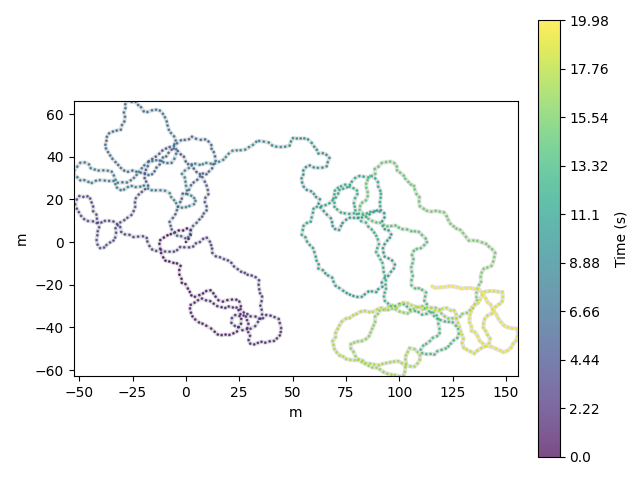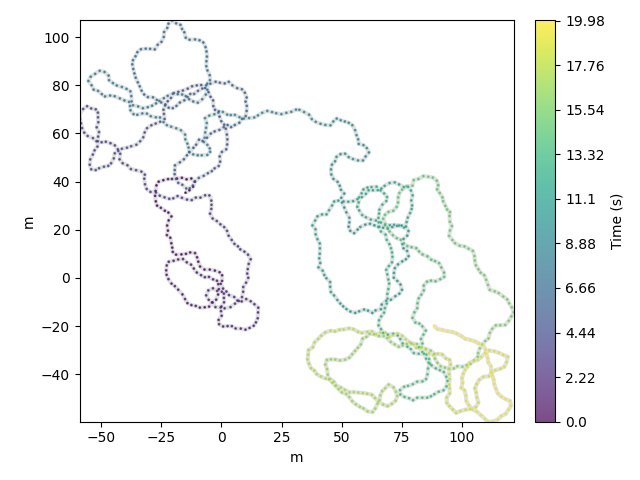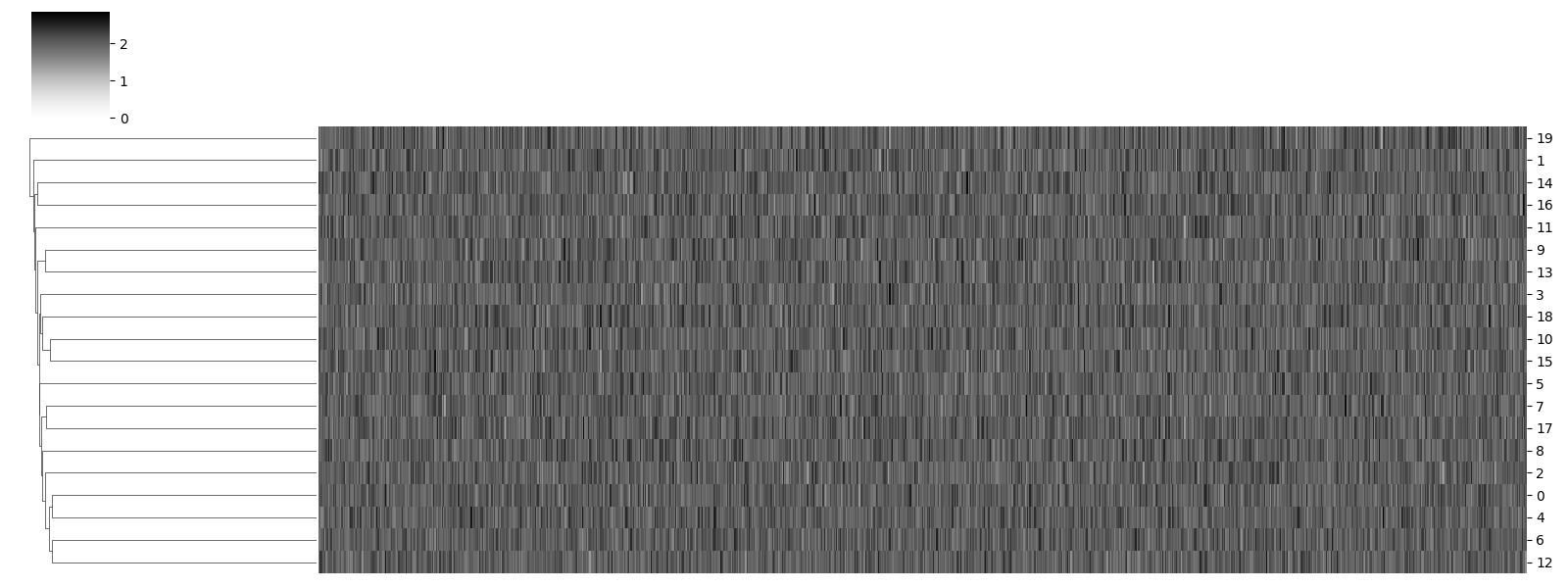# Comparing¶

traja allows comparing trajectories using various methods.

```import traja

df = traja.generate(seed=0)
df.traja.plot()
``````<matplotlib.collections.PathCollection object at 0x7f3c157b0110>
```

## Fast Dynamic Time Warping of Trajectories¶

Fast dynamic time warping can be performed using `fastdtw`. Source article: link.

```import numpy as np

rotated = traja.rotate(df, angle=np.pi / 10)
rotated.traja.plot()
``````<matplotlib.collections.PathCollection object at 0x7f3b98c2d450>
```

## Compare trajectories hierarchically¶

Hierarchical agglomerative clustering allows comparing trajectories as actograms and finding nearest neighbors. This is useful for comparing circadian rhythms, for example.

```# Generate random trajectories
trjs = [traja.generate(seed=i) for i in range(20)]

# Calculate displacement
displacements = [trj.traja.calc_displacement() for trj in trjs]

traja.plot_clustermap(displacements)
``````/home/docs/checkouts/readthedocs.org/user_builds/traja/envs/latest/lib/python3.7/site-packages/seaborn/matrix.py:657: UserWarning: Clustering large matrix with scipy. Installing `fastcluster` may give better performance.
warnings.warn(msg)

<seaborn.matrix.ClusterGrid object at 0x7f3ba9c31050>
```

## Compare trajectories point-wise¶

```dist = traja.distance_between(df.traja.xy, rotated.traja.xy)

print(f"Distance between the two trajectories is {dist}")
```
```Distance between the two trajectories is 27254.161638114736
```

Total running time of the script: ( 0 minutes 1.392 seconds)

Gallery generated by Sphinx-Gallery